Courses
Courses for Kids
Free study material
Free LIVE classes
More

# Carbohydrates JEE Advanced 2023 Revision Notes

Last updated date: 30th Mar 2023
Total views: 85.8k
Views today: 0.62k

Carbohydrates are one type of organic compound that constitute a lot of substances we find in our daily life. This class of organic compounds is one of the four major bio-molecules found in organisms. It is made of carbon, hydrogen, and oxygen and has a general formula of (CH2O)n. It is one of the biggest classifications of biomolecules that is explained with formula and constituent functional groups. To understand the concepts of this classification of bio-molecules, download and refer to the Carbohydrates JEE Advancedfor free from Vedantu.

These notes have been prepared by our subject-matter experts focusing on the major features of this biomolecule. These notes explain the structural classification of carbohydrates along with a proper illustration of their constituent elements. To make it easier to revise the concepts, refer to these revision notes available on Vedantu.

## Access JEE Advanced Important Questions Chemistry Carbohydrates

### Single Choice Type Question

1. The structure of D-(+)-glucose is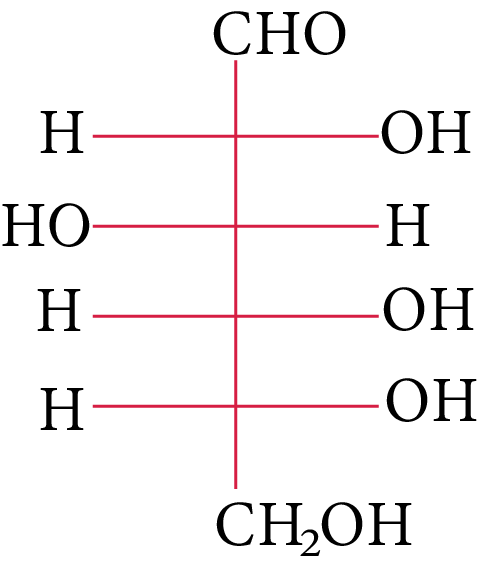D-(+)-glucose

The structure of L-(−)-glucose is

(A)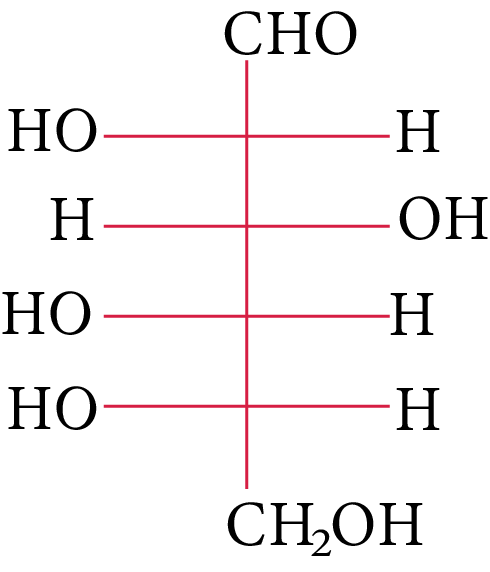L-(-)-glucose

(B)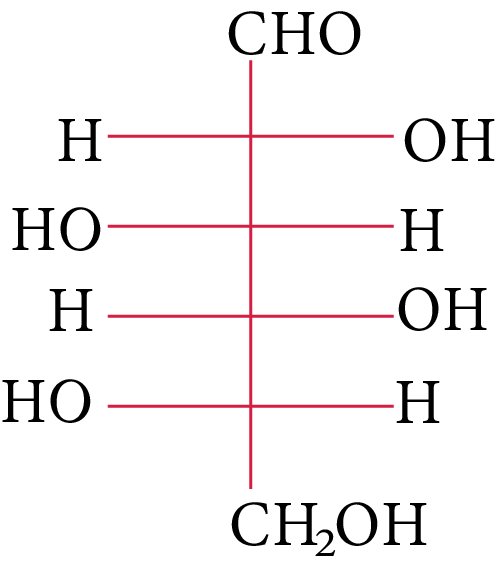D-(+)-maltose

(C)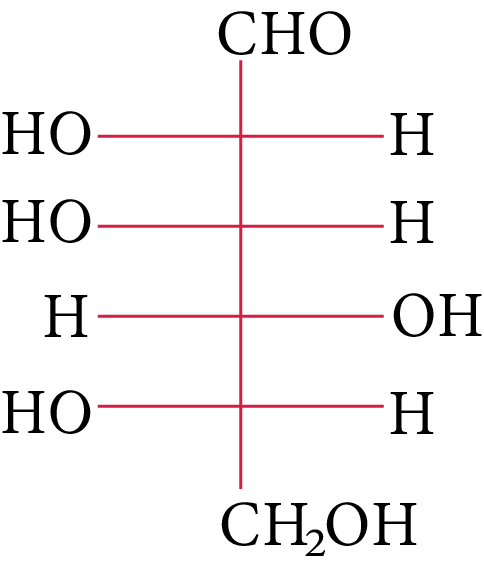D-(+)-lactose

(D)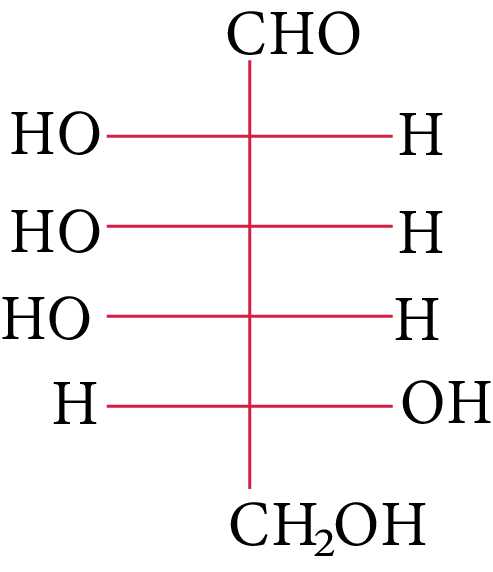D-(+)-galactose

1. The following carbohydrate is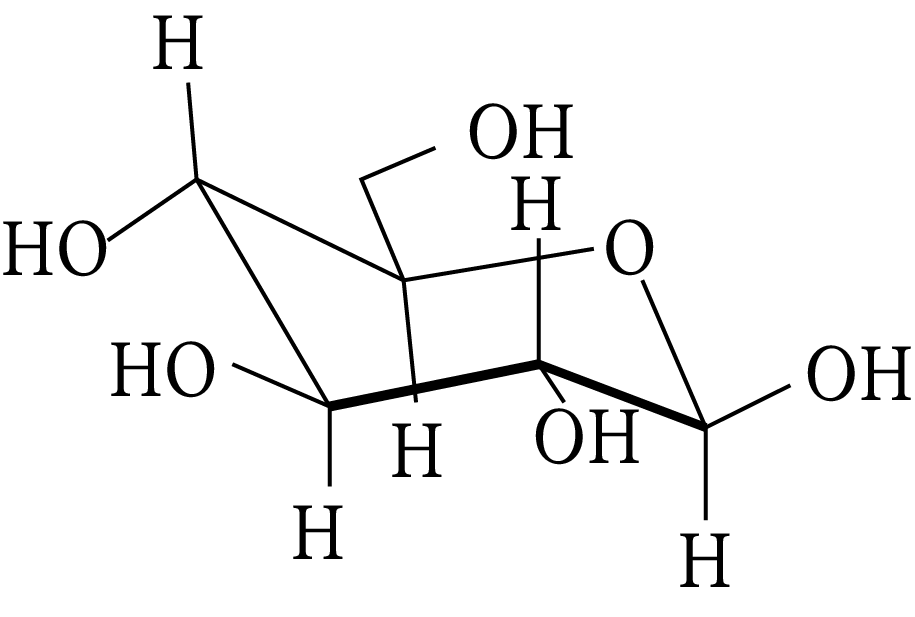Carbohydrate

JEE 2011

(A) a ketohexose

(B) an aldohexose

(C) an alpha furanose

(D) an alpha pyranose

1. The correct statement about the following disaccharide is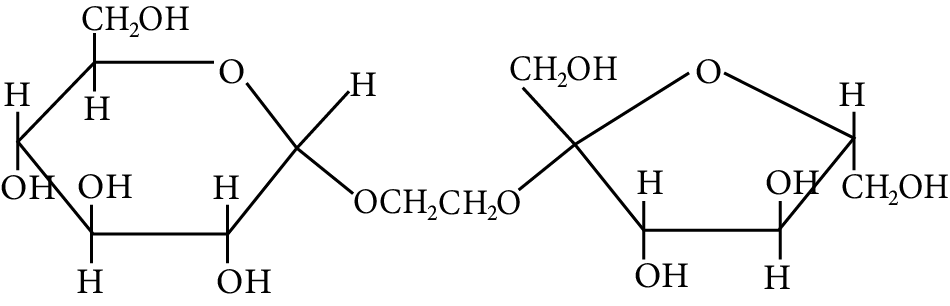Disaccharide

IIT 2010

(A) Ring (A) is pyranose with $\alpha$  glycosidic link

(B) Ring (A) is furanose with $\alpha$  glycosidic link

(C) Ring (B) is furanose with $\alpha$  glycosidic link

(D) Ring (B) is pyranose with $\beta$ -glycosidic link

1. The Fischer presentation of D-glucose is given below.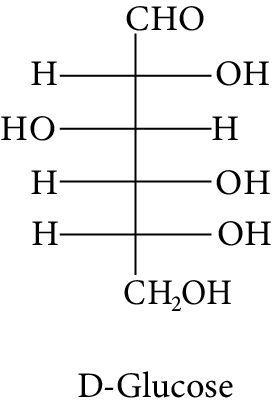D-Glucose

The correct structure (s) of beta glucopyranose is (are):

(A)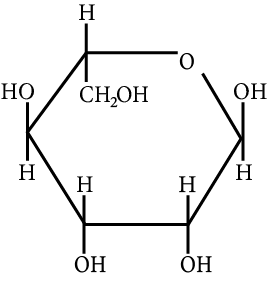Carbohydrate

(B)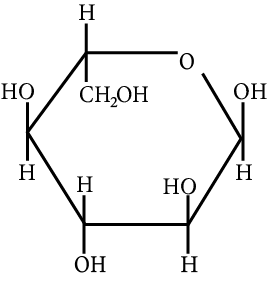Carbohydrate

(C)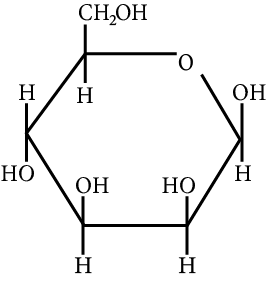Carbohydrate

(D)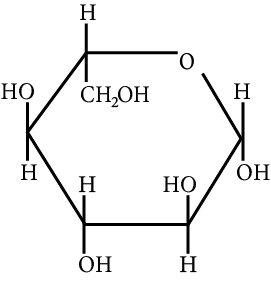Beta Glucopyranose

1. The change in the optical rotation of freshly prepared solution of glucose is known as

AIEEE 2011

(A) tautomerism

(B) racemization

(C) specific rotation

(D) mutarotation

1. Accumulation of which of the following molecules in the muscles occurs as a result of vigorous exercise?

(A) Pyruvic acid

(B) L-lactic acid

(C) Glycogen

(D) Glucose

1. Which compounds will behave as reducing sugar in an aqueous KOH solution?

Different Types of Carbohydrate

1. Complete hydrolysis of starch gives:

(A) glucose and fructose in equimolar amounts

(B) glucose only

(C) galactose and fructose in equimolar amounts

(D) glucose and galactose in equimolar amounts

1. Glycogen is a branched chain polymer of $\alpha - D - {\rm{glucose}}$  units in which chain is formed by ${{\rm{C}}_1}{\rm{ }} - {\rm{ }}{{\rm{C}}_4}$  glycosidic linkage whereas branching occurs by the formation of ${{\rm{C}}_1}{\rm{ }} - {\rm{ }}{{\rm{C}}_6}$  glycosidic linkage. Structure of glycogen is similar to __________.

(A) Amylose

(B) Amylopectin

(C) Cellulose

(D) Glucose

1. Sucrose (cane sugar) is a disaccharide. One molecule of sucrose on hydrolysis gives _________.

(A) 2 molecules of glucose

(B) 2 molecules of glucose $$+$$ 1 molecule of fructose

(C) 1 molecule of glucose $$+$$ 1 molecule of fructose

(D) 2 molecules of fructose

1. Which of the following pairs represents anomers?

(A)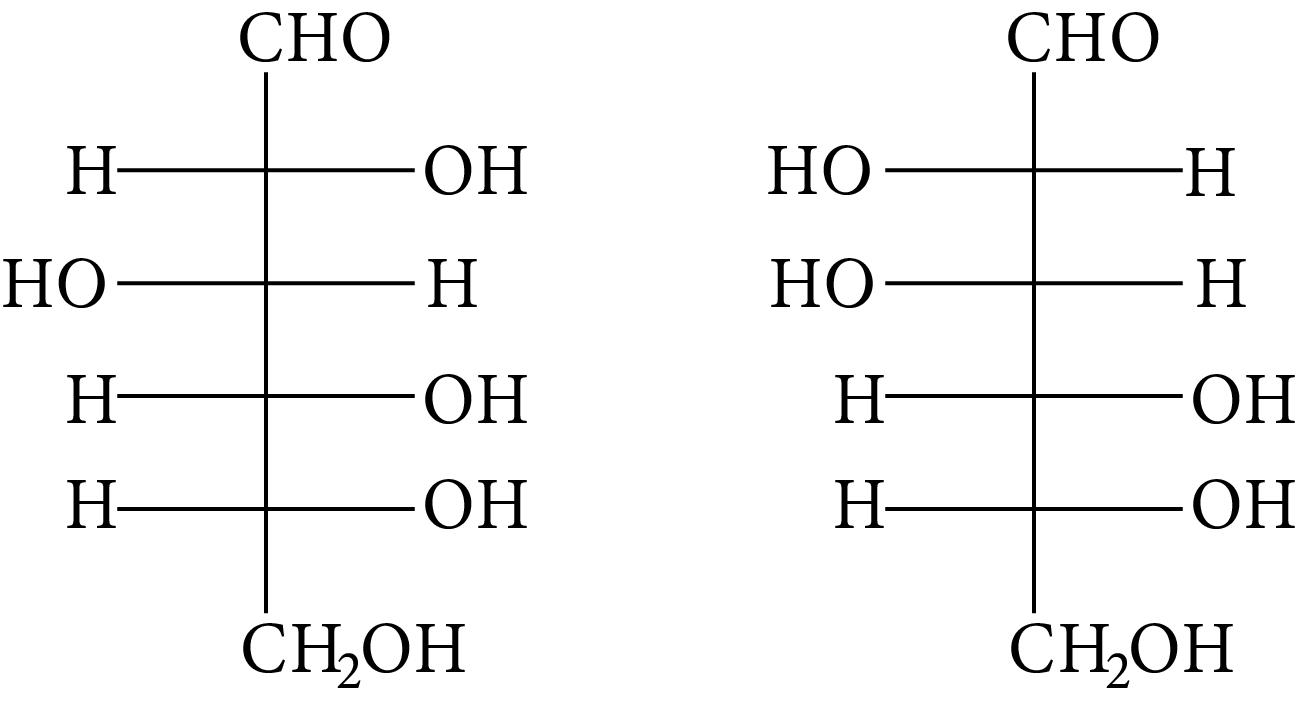(B)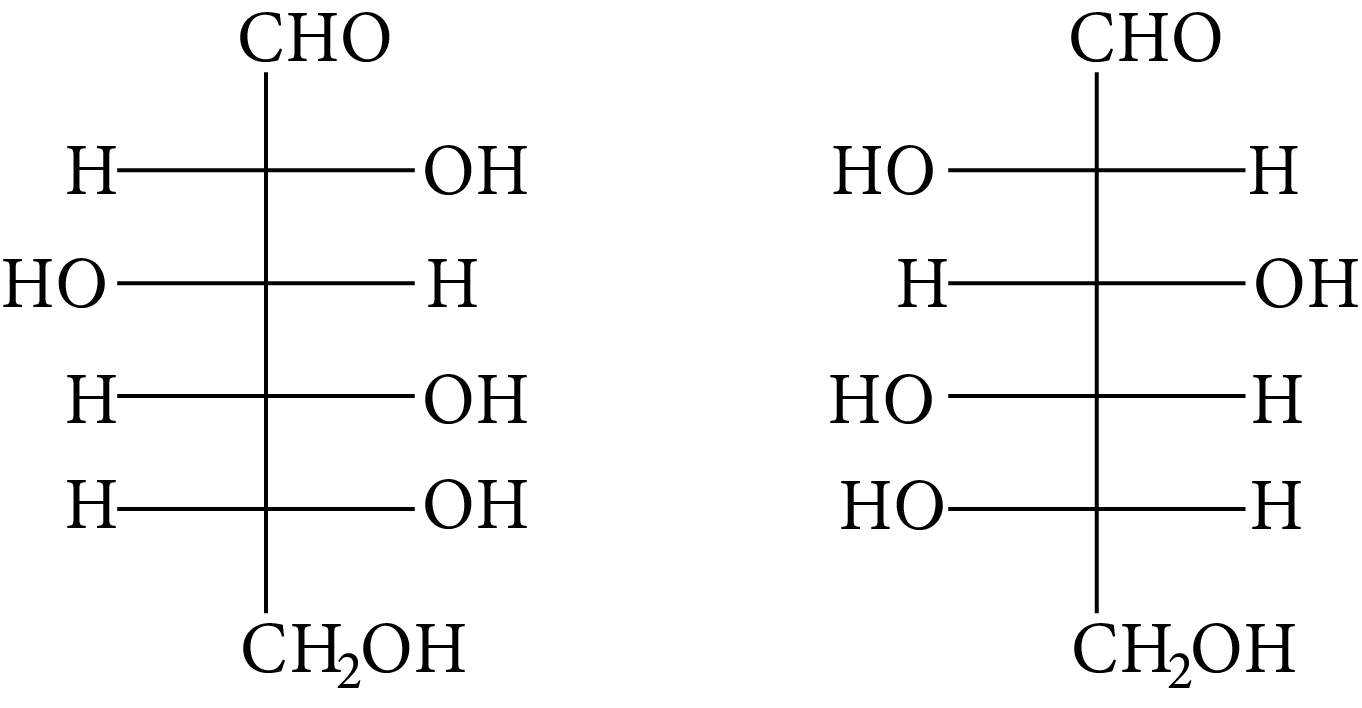(C)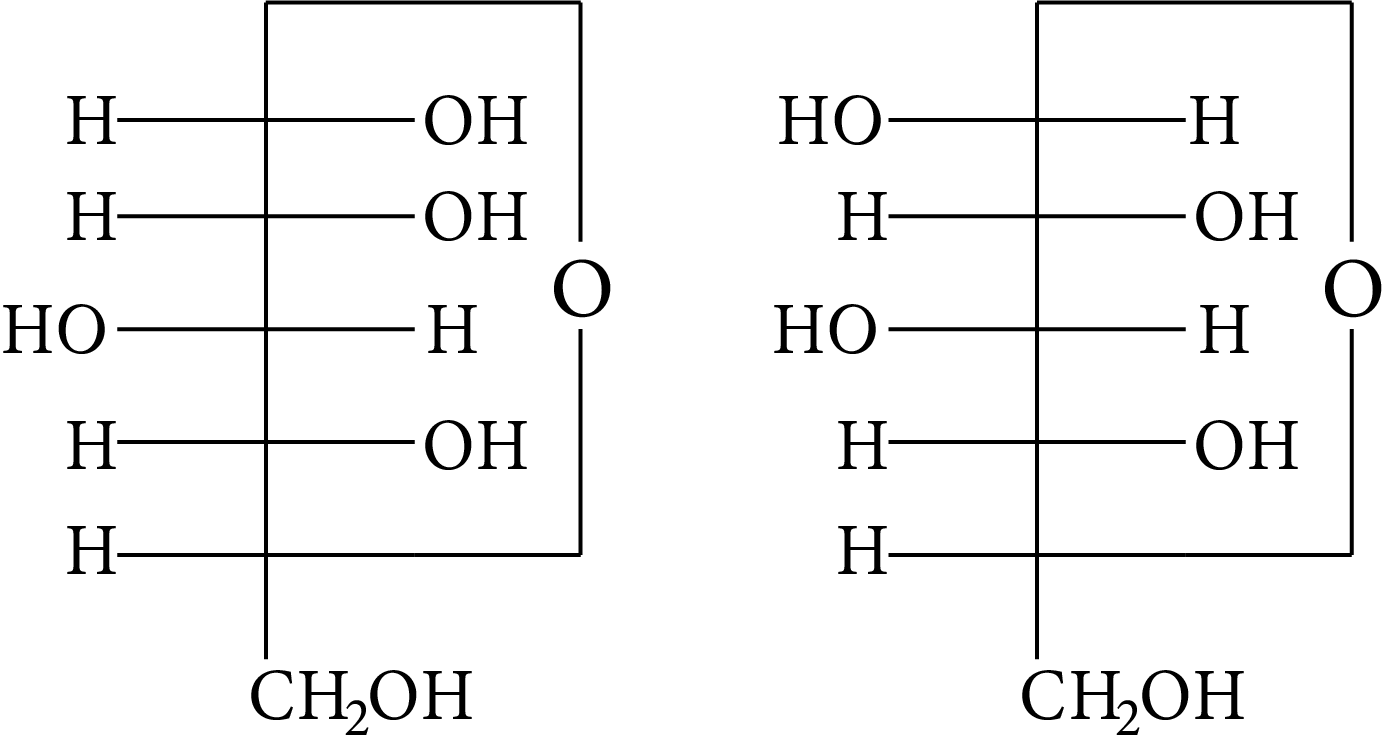(D)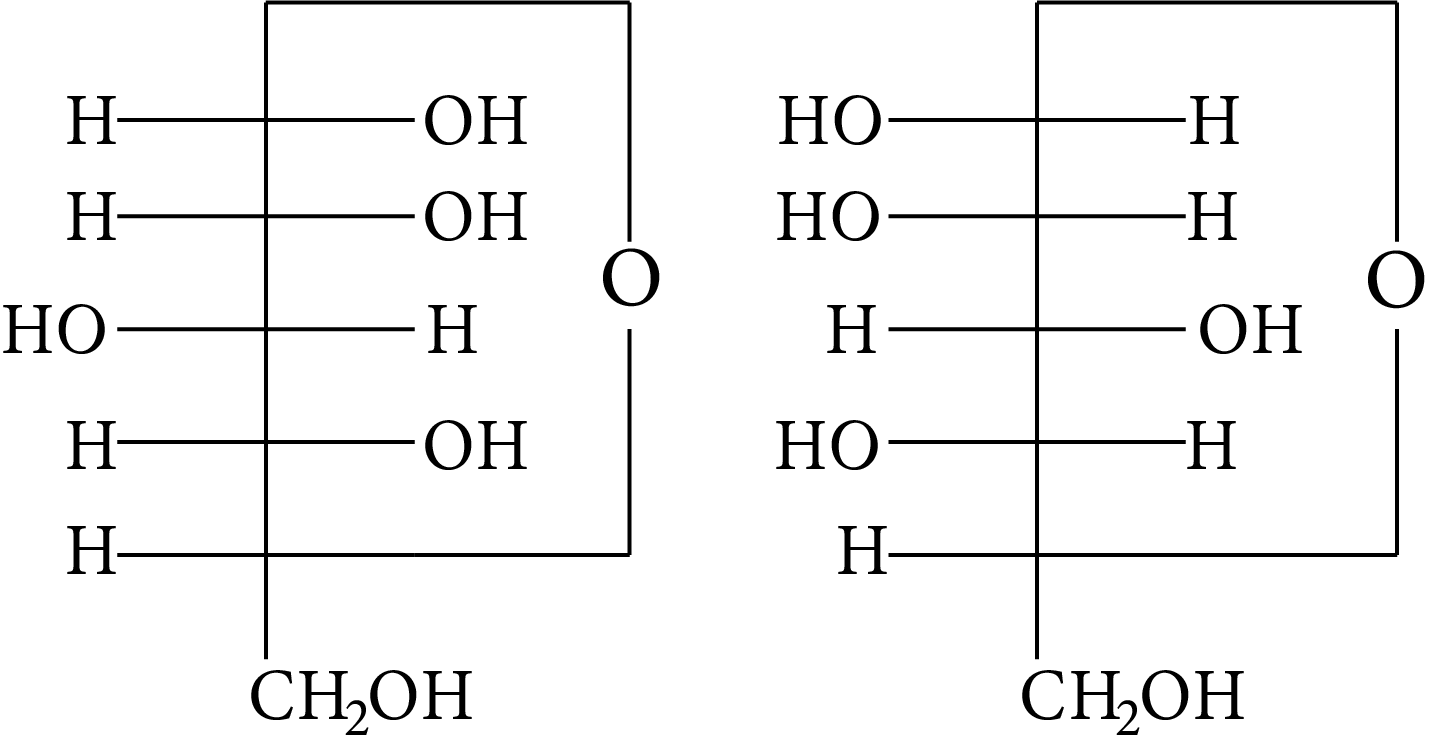1. In disaccharides, if the reducing groups of monosaccharides i.e. aldehydic or ketonic groups are bonded, these are non-reducing sugars. Which of the following disaccharide is a non-reducing sugar?

(A)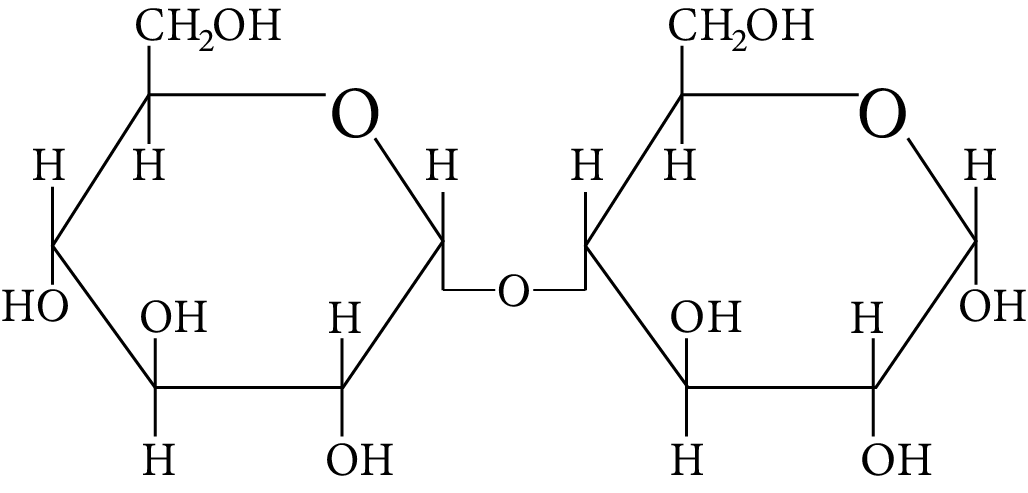Disaccharide

(B)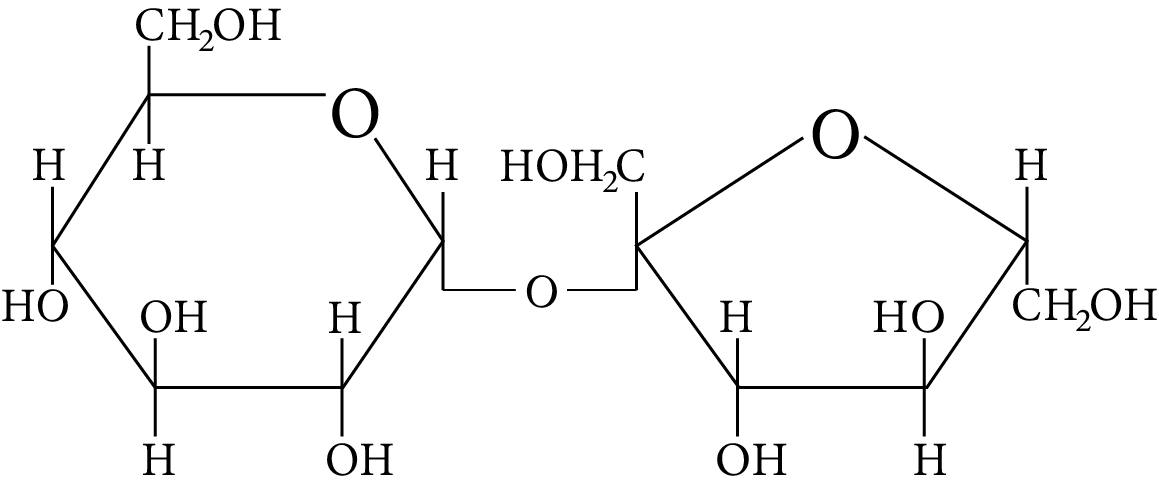Disaccharide

(C)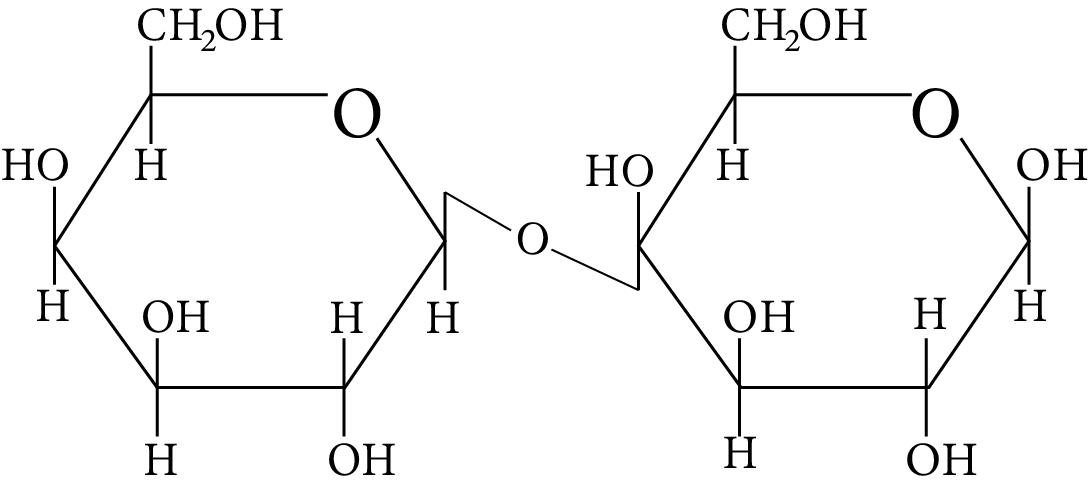Disaccharide

(D)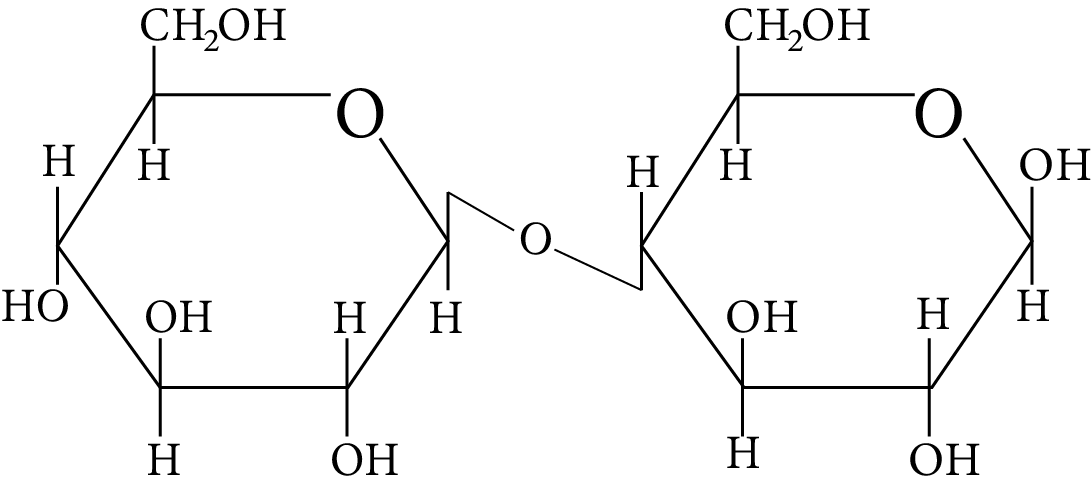Disaccharide

1.  Two nucleotides are joined by phosphodiester linkage to form a dinucleotide. Which pentose sugars and nucleotides' carbon atoms are connected by these linkages?

(A) $5\prime {\rm{ and }}3\prime$

(B) $1\prime {\rm{ and }}5\prime$

(C) $5\prime {\rm{ and }}5\prime$

(D) $3\prime {\rm{ and }}3\prime$

## Multiple Choice Type Question

1. Which of the following statements is not true about glucose?

(A) It is an aldohexose.

(B) On heating with ${\rm{HI}}$  it forms n-hexane.

(C) It is present in furanose form.

(D) It does not give $2,4 - {\rm{DNP}}$  test.

1. Carbohydrates are classified on the basis of their behaviour on hydrolysis and also as reducing or non- reducing sugar. Sucrose is a __________.

(A) monosaccharide

(B) disaccharide

(C) reducing sugar

(D) non-reducing sugar

1. Which of the following carbohydrates are branched polymers of glucose?

(A) Amylose

(B) Amylopectin

(C) Cellulose

(D) Glycogen

1. Which of the following statement(s) is(are) true?

(A) The two six-membered cyclic hemiacetal forms of D-(+)-glucose are called anomers

(B) Oxidation of glucose with bromine water gives glutamic acid

(C) Monosaccharides cannot be hydrolyzed to given polyhydroxy aldehydes and ketones

(D) Hydrolysis of sucrose gives dextrorotatory glucose and laevorotatory fructose

1. For "invert sugar", the correct statement(s) is(are)

(Given : specific rotations of (+)-sucrose, (+)-maltose, L-(−)-glucose and L-(+)-fructose in aqueous solution are $+66^{\circ},+140^{\circ},-52^{\circ} \text { and }+92^{\circ},$  respectively.)

(A) “Invert sugar” is prepared by acid catalysed hydrolysis of maltose.

(B) “Invert sugar” is an equimolar mixture of D-(+)-glucose and D-(+)-fructose.

(C) Specific rotation of “invert sugar” is -20.

(D) In reaction with Br2 water, “invert sugar” forms saccharic acid as one of the products.

1. Given: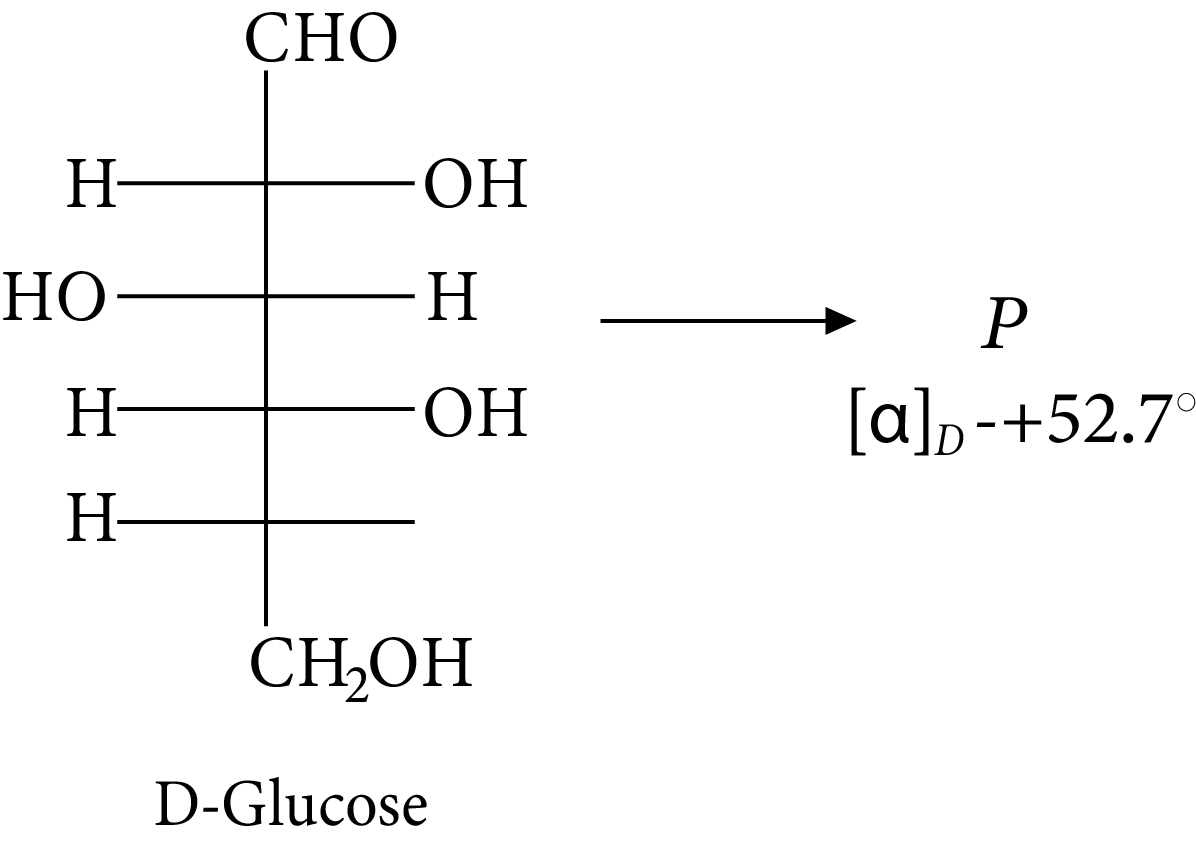D-glucose

The compound(s), which on reaction with HNO3 will give the product having degree of rotation,${[\alpha ]_D} = - {52.7^ \circ }$  is (are)

(A)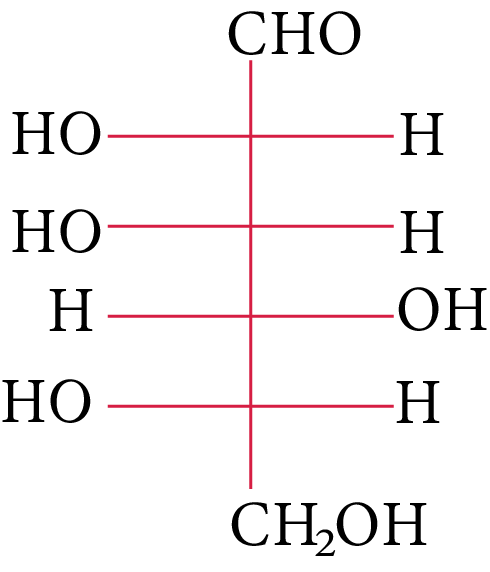(B)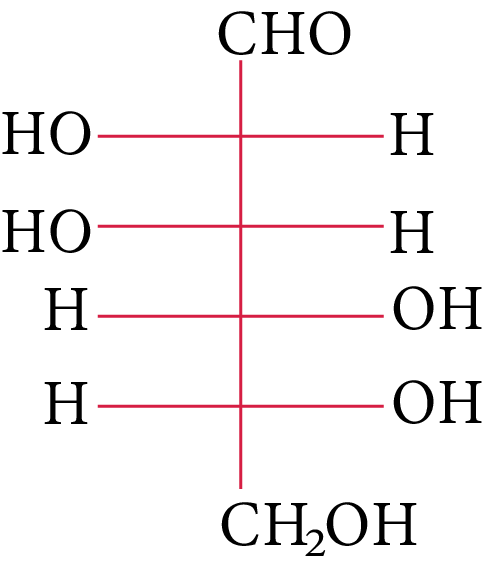(C)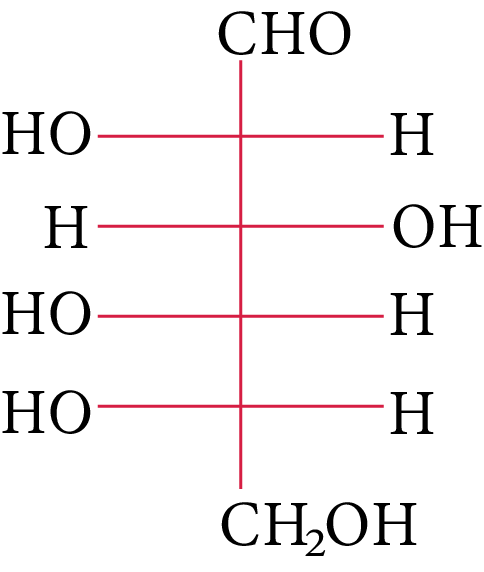(D) None of these

1. The two functional groups present in a typical carbohydrate are:

(A) $> {\rm{C}} = {\rm{O and - OH}}$

(B) ${\rm{ - OH and - CHO}}$

(C) ${\rm{ - OH and - COOH}}$

(D) ${\rm{ - CHO and - COOH}}$

1. Consider the following statements about glucose and state which of the following are correct.

(1) The glucose reaction with hydroxylamine and HCN confirms the presence of a carbonyl group in glucose.

(2) The reaction of glucose with nitric acid confirms the presence of a primary alcoholic group in glucose.

(3) The glucose reaction with HI suggests that all six carbon atoms are connected in a straight chain.

(A) (1)

(B) (2)

(C) (3)

(D) Only (1)

1. Consider the following carbohydrates.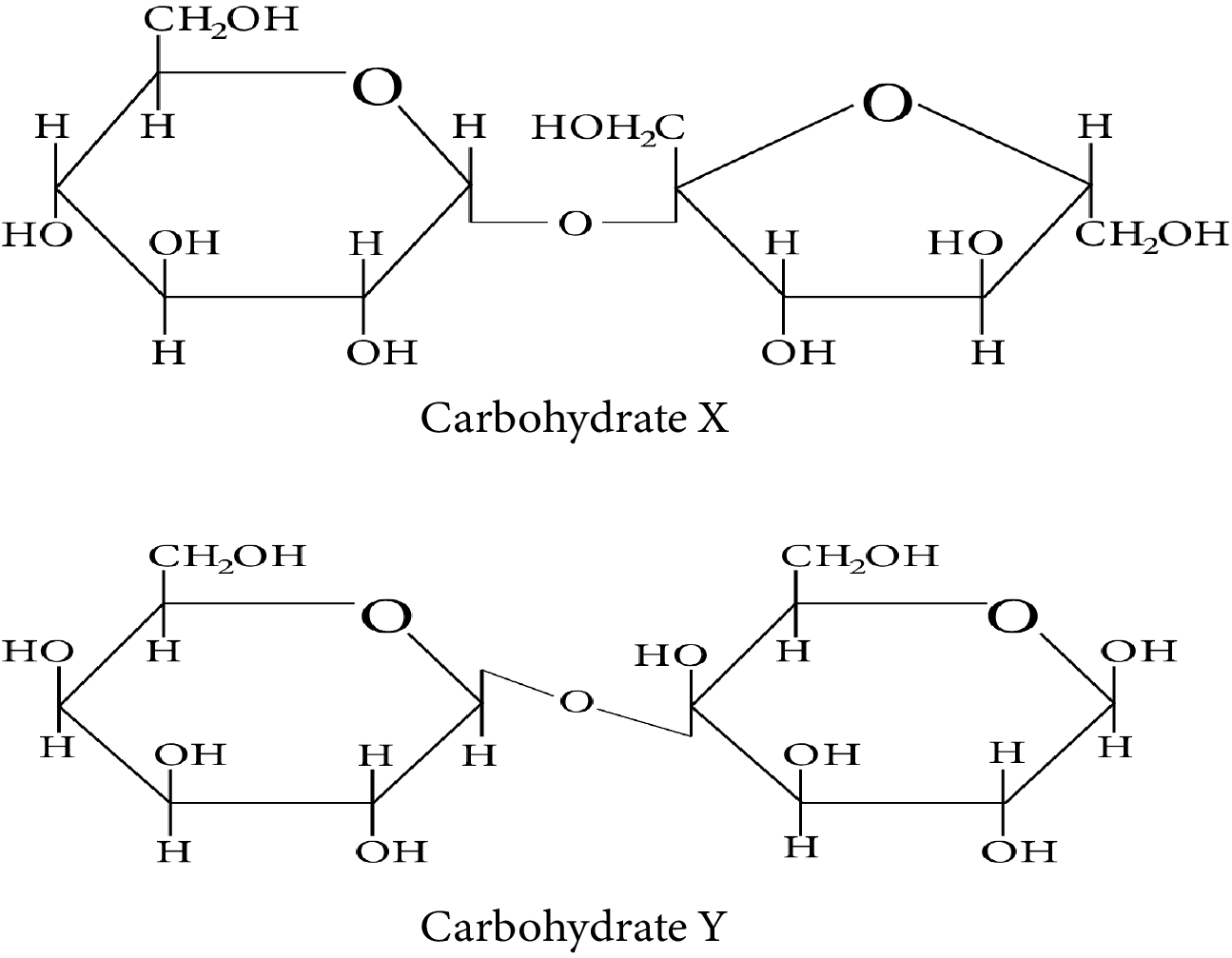Carbohydrate X and Y

Which of the following statement(s) is/are true regarding the two carbohydrates:

(i) X is a reducing sugar and Y is a non-reducing sugar.

(ii) X has ${\rm{\alpha }} - 1{\rm{, \beta }} - 2$  glycosidic linkage whereas Y has ${\rm{\beta }} - 1,4$  glycosidic linkage.

(iii) On treatment with sulfuric acid, X gives elemental carbon.

(iv) Y can have 4 anomeric forms.

(A) (i)

(B) (ii)

(C) (iii)

(D) (iv)

## Integer Type Questions

1. When the following aldohexose exists in its d-configuration, the total number of stereoisomers in its pyranose form is

1.  The number of chiral carbons present in sucrose is _____.

JEE Main - 2020

1. Synthesis of each molecule of glucose in photosynthesis involves:

### Solutions

1. (A)

The OH group linked to the final stereocenter is on the right side of D-(+)-glucose while it is on the left side of L-(-)-glucose. The L-(-)-glucose molecule isL-(-)-glucose

1. (B)

The carbohydrate is hexose because it has six carbons. Additionally, the first carbon, anomeric carbon, contains hydrogen. The new chiral centre created by cyclization is called anomeric C (first carbon).

It is the cyclic form of D-glucose, and as glucose functions as an aldehyde, it is also known as aldohexose.

1. (A)

Pyranose is the name given to the six-membered cyclic ether, whereas furanose is the name given to the five-membered cyclic ether.

As a result, ring (a) is a pyranose and possesses an ether linkage, also known as $\alpha$ -glycosidic bond in carbohydrate chemistry, at the $\alpha$ -position.

1. (D)

The energy source D-glucopyranose, a synthetic simple monosaccharide, has a beta isoform called beta-D-glucopyranose.

1. (D)

The freshly generated glucose solution equilibrium results in an uneven combination of (+) and (-) glucose. This occurrence is known as mutarotation.

1. (B)

Exercise that is intense causes L-lactic acid to build up in the muscles.

Anaerobic respiration, or the breakdown of carbohydrates in the absence of oxygen, is to blame.

1. (A)

The following reaction occurs when ester is present in an aqueous KOH solution and undergoes the SNAE reaction.

A hydroxy carbonyl compound is created in the aforementioned chemical when aq. KOH (SNAE) reaction occurs, which results in a positive Tollens test result. As a result, this substance behaves like a reducing sugar in an aqueous KOH solution.

1. (B)

The monomer of starch, which is glucose, is a combination of the polysaccharides amylose and amylopectin. So, upon total hydrolysis, it solely yields glucose.

1. (B)

Glycogen is similar to the structure of amylopectin. It is an $\alpha - D - {\rm{glucose}}$  unit branched chain polymer which is created by the glycosidic linkage of ${{\rm{C}}_1}{\rm{ }} - {\rm{ }}{{\rm{C}}_4}$  and branching by ${{\rm{C}}_1}{\rm{ }} - {\rm{ }}{{\rm{C}}_6}$  glycosidic linkage.

Thus, option (B) is correct.

1. (C)

Sucrose (also known as cane sugar) is a type of disaccharide. When sucrose is hydrolyzed, one molecule of glucose and one molecule of fructose are produced.

1. (C)

At ${\rm{C}} - 1,$  anomers have a distinct configuration. If hydroxyl group is present on the right side of anomeric carbon, it is referred to as the $\alpha -$ form whereas if hydroxyl group is present on the left side, it is referred to as the $\beta -$ form. Therefore, among all the given pairs, option (C) represents anomers.

1. (B)

Among all the given disaccharides, the structure given in option (B) represents sucrose, in which $\alpha - D -$  glucose and $\beta - D -$ fructose are linked by a ${{\rm{C}}_1} - {{\rm{C}}_2}$  glycosidic bond.

Due to the involvement of the reducing groups of glucose and fructose in the formation of glycosidic bonds, this is classified as a non-reducing sugar.Sucrose

Thus, option (B) is correct.

1. (A)

The two nucleotides are linked together by a phosphodiester to form a dinucleotide. The pentose sugars of nucleotides have 5′ and 3′ linkages. Thus, option (A) is correct.

1. (A, and B)

Glucose exists as pyranose, which has a five-membered ring structure. As a result, the statement in option (C) is incorrect.

Glucose is a monosaccharide containing six carbon atoms and an aldehyde group. As a result, it's a type of aldohexose. As a result, the statement in option (A) is correct.

When glucose is heated with, n-hexane is produced. Due to this process, all six carbon atoms in glucose are bonded together in a straight chain. As a result, the statement in option (B) is correct.

The aldehyde group is not free in the cyclic structure. As a result, it does not offer a 2-4 DNP test. Thus, the statement given in option (D) is correct.

1. (B and D)

Sucrose is a common disaccharide which on hydrolysis produces an equimolar combination of $D - \left( + \right) -$ glucose and $D - \left( - \right) -$ fructose. A glycosidic link between C1 of $\alpha -$ glucose and C2 of $\beta -$ fructose holds these two monosaccharides together. Because the reducing groups of glucose and fructose are involved in forming glycosidic bonds, sucrose is a non-reducing sugar. Thus, options (B) and (D) are correct.

1. (B and D)

Amylopectin and Glycogen are the correct choices because they are types of carbohydrate storage in animal tissues, whereas amylose is made up of $80 - 85{\rm{ \% }}$  starch. It is also a branched polymer of glucose that is linked together via ${\rm{C1}} - {\rm{C6}}$ glycosidic linkage.

Thus, options (B) and (D) are correct.

1. (A, C and D)

(A) Anomers are two six-membered cyclic hemiacetal forms of D-(+)-glucose. Both are anomers.
(B) Gluconic acid is produced when glucose is oxidised in the presence of ${\rm{B}}{{\rm{r}}_{\rm{2}}}$  water.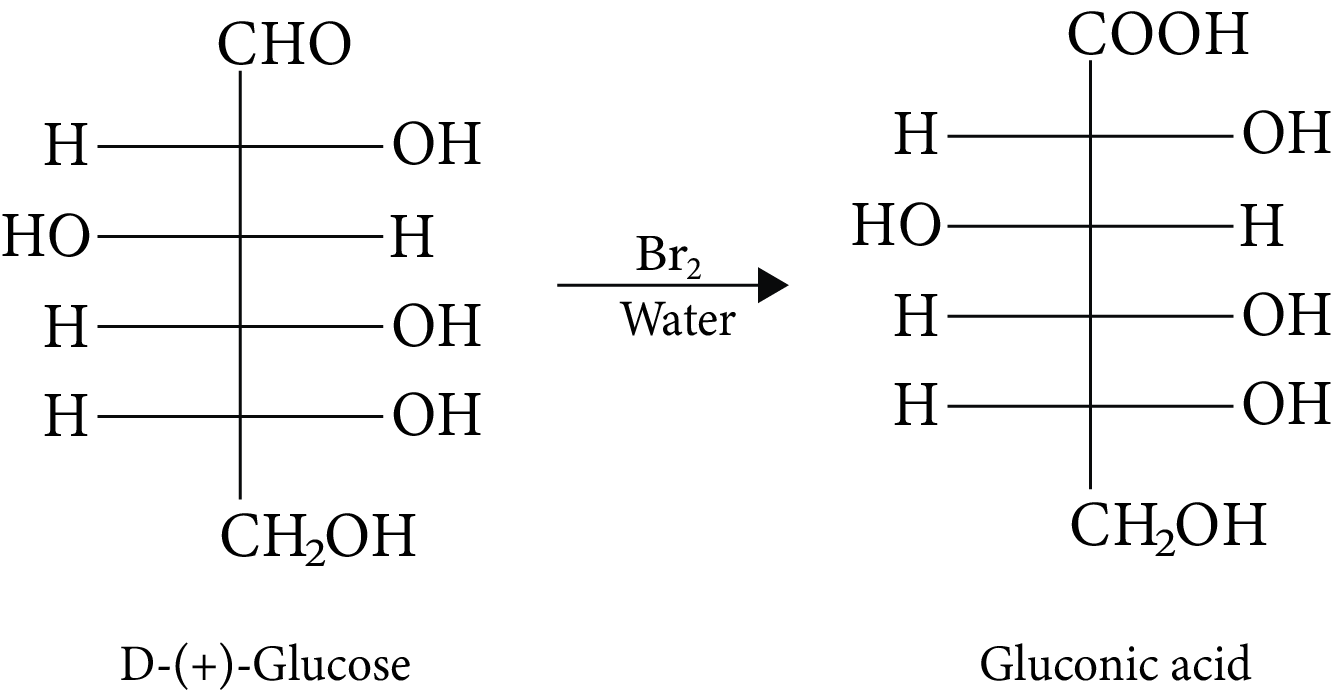D-(+)-Glucose and Gluconic acid

(C) It is impossible to hydrolyze monosaccharides into polyhydroxy aldehydes and ketones.

(D) D-glucose and L-fructose are produced during sucrose hydrolysis.

1. (B and C)

The hydrolysis of sucrose under acid catalysis produces invert sugar.

The specific rotation of invert sugar is $\begin{array}{c}{\left[ \alpha \right]_{{\rm{mix}}}} = 0.5 \times \left( { + 52} \right) + 0.5 \times \left( { - 92} \right)\\ = + {\rm{ }}26 - 46{\rm{ }}\\ = - {20^ \circ }\end{array}$

When invert sugar reacts with ${\rm{B}}{{\rm{r}}_{\rm{2}}}$  water, one of the results is gluconic acid. Fructose is not oxidised by ${\rm{B}}{{\rm{r}}_{\rm{2}}}$  water, which converts glucose into gluconic acid.

1. (c)

In its reaction with ${\rm{HN}}{{\rm{O}}_{\rm{3}}}{\rm{,}}$  monosaccharide oxidised to produce carboxylic acids. Along with aldehyde, primary alcohols also oxidise to carboxylic acid.

Rotation of the product (P) enantiomer is ${\rm{52}}{\rm{.}}{{\rm{7}}^{\rm{0}}}{\rm{.}}$

1. (A and B)

Carbohydrates are polyhydroxy aldehydes, polyhydroxy ketones, or substances that, upon hydrolysis, provide both aldehyde and ketonic groups. As a result, (carbonyl) aldehyde, ketone, and hydroxyl groups are the main functional groups.

1. (A, B and C)

Glucose is one of the important carbohydrates with the molecular formula of ${{\rm{C}}_{\rm{6}}}{{\rm{H}}_{{\rm{12}}}}{{\rm{O}}_{\rm{6}}}.$  It is also referred as aldohexose because it contains six carbon atoms and an aldehyde group.

When glucose is treated with hydroxylamine, an oxime is formed. When glucose is treated with hydrogen cyanide, cyanohydrin is formed.

These two statements suggest that glucose contains a carbonyl group.

Thus, statement (1) is correct.

Glucose gets oxidised in the presence of nitric acid and results in the formation of saccharic acid.

Here, the number of carbon atoms remains the same, which shows the presence of the primary alcoholic group in glucose.

Thus, statement (2) is also correct.

Glucose on prolonged heating with HI and red phosphorus yields n-hexane.

This statement suggests that all six carbon atoms are linked in a straight chain.

Thus, statement (3) is also correct.

1. (B and C)

Compound X contains two subunits, glucose and fructose. Therefore, X is sucrose which is one of the common disaccharides. On hydrolysis, it gives equimolar mixture of $D - \left( - \right){\rm{fructose}}$  and $D - \left( + \right){\rm{glucose}}{\rm{.}}$

The two monosaccharides, glucose and fructose, are joined by glycosidic linkage between C-1 of ${\rm{\alpha }} - D - {\rm{glucose}}$  and C-2 of ${\rm{\beta }} - D - {\rm{fructose}}{\rm{.}}$

Since the reducing groups of fructose and glucose are involved in the formation of glycosidic bonds, the sugar is non-reducing in nature. Therefore, carbohydrate X is a non-reducing sugar.

Compound Y contains two subunits, galactose and glucose. Therefore, Y is Lactose.

Lactose is a disaccharide which is found in milk. It is also known as milk sugar. It consists of glucose and galactose subunits, joined by glycosidic linkage between C-1 of ${\rm{\beta }} - D - {\rm{galactose}}$  and C-4 of ${\rm{\beta }} - D - {\rm{glucose}}{\rm{.}}$

A free aldehyde group may be formed in Lactose at C-1 of the glucose unit. Hence, this sugar is reduced in nature. Therefore, carbohydrate Y is a reducing sugar.

The systematic name of Lactose is ${\rm{\beta }} - D - {\rm{galactopyranosyl}} - \left( {1 \to 4} \right) - D - {\rm{glucose}}{\rm{.}}$  The glucose can have ${\rm{\alpha }} - {\rm{pyronose}}$  and ${\rm{\beta }} - {\rm{pyronose}}$  forms whereas the galactose can only have ${\rm{\beta }} - {\rm{pyronose}}$  form. Hence, ${\rm{\alpha }} - {\rm{Lactose}}$  and ${\rm{\beta }} - {\rm{Lactose}}$  are two possible anomeric forms of glucopyranose ring alone.

1. 8

The three chiral carbon configurations (starting) can be altered while retaining the d-configuration. Consequently, the total number of d-pyranose stereoisomers.

So, 8 is the correct answer.

1. 9

A carbon atom that is joined to four different kinds of atoms or groups of atoms is said to be chiral. So, the total number of chiral carbons present in sucrose is 9.

1. 18

$\begin{array}{*{20}{l}}{{\rm{12}}{{\rm{H}}_{\rm{2}}}{\rm{O + 12 NADP + 18 ADP}} \to {\rm{6}}{{\rm{O}}_{\rm{2}}}{\rm{ + 18 ATP + 12 NADPH }}\left( {{\rm{ light reaction}}} \right)}\\{{\rm{6C}}{{\rm{O}}_{\rm{2}}}{\rm{ + 12 NADPH + 18 ATP}} \to {{\rm{C}}_{\rm{6}}}{{\rm{H}}_{{\rm{12}}}}{{\rm{O}}_{\rm{6}}}{\rm{ + 12 NADP + 18 ADP + 6}}{{\rm{H}}_{\rm{2}}}{\rm{O }}\left( {{\rm{dark reaction}}} \right)}\\{{\rm{6C}}{{\rm{O}}_{\rm{2}}}{\rm{ + 6}}{{\rm{H}}_{\rm{2}}}{\rm{O}} \to {{\rm{C}}_{\rm{6}}}{{\rm{H}}_{{\rm{12}}}}{{\rm{O}}_{\rm{6}}}{\rm{ + 6}}{{\rm{O}}_{\rm{2}}}{\rm{}}\left( {{\rm{Net reaction}}} \right)}\end{array}$

So, 18 molecules of ATP are involved in synthesising each molecule of glucose in photosynthesis.

## Importance of Carbohydrates

This chapter explains the formula of carbohydrates and what are they made of. The constituent elements are explained in the form of chains and the bonds between them. Carbohydrates can contain ketone, aldehyde, and poly-hydroxy functional groups.

These compounds are present in various living organisms. The term carbohydrates is introduced to students in several preliminary concepts like photosynthesis where the formula of glucose is also introduced. This chapter will proceed to explain how these carbohydrate molecules are identified and distinguished into various types.

The explanation of the formation of polymeric chains in carbohydrates also explains their complex forms in plants and animals. This chapter explains the difference between carbohydrates with the same constituent atoms and formulas.

This chapter is very important for the preparation for the JEE Advanced exam. It introduces this biomolecule in a broader way. The foundation of this chapter will be used in other chapters of this subject and even in other subjects too.

## Benefits of Carbohydrates JEE Advanced Notes PDF

• These revision notes have been compiled with the sole motive to explain the concepts in a simpler format. You will learn these fundamental concepts without facing any difficulty and will be able to revise them in no time with the help of these notes.

• Once you have completed the chapter, use these notes to go through the concepts for revision. The easier format followed in compiling the notes will also guide students to remember what they have studied before effectively.

• The Carbohydrates JEE Advanced revision notes will support your preparation and complete the syllabus in no time. Solve the sample questions given in these notes to test your preparation level and find out where you need to focus more.

• You can learn the different uses of carbohydrates and their explanations from the experts by using these notes. Also, you will be able to learn the fundamental concepts of these complex molecules without any hassle.

Students can download the free PDF of these revision notes and prepare for the JEE Advanced exam. The simple explanation of all concepts covered in this chapter is given in a concise format so students can refer to these notes to revise this chapter quickly. They can solve the Carbohydrates JEE Advanced revision questions given in the notes to test their knowledge. Thus, these revision notes will help students to prepare this chapter well for JEE Advanced.

## FAQs on Carbohydrates JEE Advanced 2023 Revision Notes

1. What is glucose?

Glucose is a monosaccharide that contains 6 carbon atoms associated with each other and with hydrogen and oxygen atoms. It is the prime respiratory substrate prepared during photosynthesis for the generation of energy.

2. What are disaccharides?

Disaccharides are polymers of carbohydrates where only two units of monosaccharide are bonded together. The best example is sucrose. It is found in plants.

3. Name a carbohydrate found in milk.

Lactose is a disaccharide found in milk. It is also a reducing sugar by nature and is an isomeric compound of sucrose.

4. What is the common polysaccharide we find in the plant kingdom?

The most common polysaccharide found in nature is cellulose. It can be found in the cell wall of plant cells.